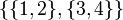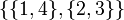Non-normal Klein four-subgroups of symmetric group:S4

This article is about a particular subgroup in a group, up to equivalence of subgroups (i.e., an isomorphism of groups that induces the corresponding isomorphism of subgroups). The subgroup is (up to isomorphism) Klein four-group and the group is (up to isomorphism) symmetric group:S4 (see subgroup structure of symmetric group:S4).
VIEW: Group-subgroup pairs with the same subgroup part | Group-subgroup pairs with the same group part | All pages on particular subgroups in groups

We consider the subgroup$H$ in the group$G$ defined as follows.$G$ is the symmetric group of degree four, which for concreteness we take as the symmetric group on the set$\{ 1,2,3,4 \}$.$H$ is the Young subgroup for the partition$\{ \{ 1,2 \} , \{ 3,4 \} \}$. Explicitly, it is the subgroup comprising those permutations that send each of the subsets$\{ 1,2 \}$ and$\{ 3,4 \}$ to within itself.$H$ has a total of three conjugates, listed below:

Our local name Partition stabilized Set of all elements in the stabilizing subgroup$\! H$$\! \{ \{ 1,2 \}, \{ 3,4 \} \}$$\! \{ (), (1,2), (3,4), (1,2)(3,4) \}$$\! H_1$$\! \{ \{ 1,3 \}, \{ 2,4 \} \}$$\! \{ (), (1,3), (2,4), (1,3)(2,4) \}$$\! H_2$$\! \{ \{ 1,4 \}, \{ 2,3 \} \}$$\! \{ (), (1,4), (2,3), (1,4)(2,3) \}$$H$ (and hence each of its conjugate subgroups) is isomorphic to the Klein four-group. However,$G$ has another subgroup isomorphic to the Klein four-group that is not one of these conjugate subgroups, and is not automorphic to these either. That is the normal Klein four-subgroup of symmetric group:S4 that comprises the identity element and the three double transpositions. The current article is not about that subgroup.

Cosets

There is a total of 18 cosets, each of which is a left coset for exactly one subgroup and a right coset for exactly one subgroup. Moreover, each coset is parametrized by the way it sends one partition (labeled) to another. Table below is incomplete.

Subset in cycle decomposition notation Subset in one-line notation Source and target subsets of$\{ 1,2,3,4 \}$ Left coset of$H$? Left coset of$H_1$? Left coset of$H_2$? Right coset of$H$? Right coset of$H_1$? Right coset of$H_2$?$\{ (), (1,2), (3,4), (1,2)(3,4) \}$ 1234, 2134, 1243, 2143$\{ 1,2 \} \to \{ 1,2 \}$,$\{ 3,4 \} \to \{ 3,4 \}$ Yes No No Yes No No$\{ (), (1,3), (2,4), (1,3)(2,4) \}$ 1234, 3214, 1432, 3412$\{ 1,3 \} \to \{ 1,3 \}$,$\{ 2,4 \} \to \{ 2,4 \}$ No Yes No No Yes No$\{ (), (1,4), (2,3), (1,4)(2,3) \}$ 1234, 4231, 1324, 4321$\{ 1,4 \} \to \{ 1,4 \}$,$\{ 2,3 \} \to \{ 2,3 \}$ No No Yes No No Yes$\{ (1,3,2,4), (1,4,2,3), (1,3)(2,4), (1,4)(2,3) \}$ 3421, 4312, 3412, 4321$\{ 1,2 \} \to \{ 3,4 \}$,$\{ 3,4 \} \to \{ 1, 2 \}$ Yes No No Yes No No$\{ (1,2,3,4), (1,4,3,2), (1,2)(3,4), (1,4)(2,3) \}$ 2341, 4123, 2143, 4321$\{ 1,3 \} \to \{ 2,4 \}$,$\{ 2,4 \} \to \{ 1,3 \}$ No Yes No No Yes No$\{ (1,2,4,3), (1,3,4,2), (1,2)(3,4), (1,3)(2,4) \}$ 2413, 3142, 2143, 3412$\{ 1,4 \} \to \{ 2,3 \}$,$\{ 2,3 \} \to \{ 1,4 \}$ No No Yes No No Yes

Complements

None of these subgroups has a permutable complement. All the subgroups do have a common lattice complement; in fact, there is a conjugacy class of subgroups each of which is conjugate to each of these subgroups. That conjugacy class is the conjugacy class of A3 in S4, which includes each of these subgroups:$\! \{ (), (1,2,3), (1,3,2) \}, \{ (), (2,3,4), (2,4,3) \}, \{ (), (1,3,4), (1,4,3) \}, \{ (), (1,2,4), (1,4,2) \}$

Properties related to complementation

Property Meaning Satisfied? Explanation Comment
retract has a normal complement No
permutably complemented subgroup has a permutable complement No
lattice-complemented subgroup has a lattice complement Yes$\{ (), (1,2,3), (1,3,2) \}$
direct factor normal subgroup with normal complement No
complemented normal subgroup normal subgroup with permutable complement No

Arithmetic functions

Function Value Explanation
order of whole group 24
order of subgroup 4
index of the subgroup 6
size of conjugacy class 3
number of conjugacy classes in automorphism class 1

Effect of subgroup operators

In the table below, we provide values specific to$H$.

Function Value as subgroup (descriptive) Value as subgroup (link) Value as group
normalizer$\{ (), (1,2), (3,4), (1,2)(3,4), (1,3)(2,4), (1,4)(2,3), (1,3,2,4), (1,4,2,3) \}$ D8 in S4 dihedral group:D8
centralizer the subgroup itself (current page) Klein four-group
normal core trivial subgroup -- trivial group
normal closure the whole group -- symmetric group:S4
characteristic core trivial subgroup -- trivial group
characteristic closure the whole group -- symmetric group:S4

Subgroup properties

Normality-related properties

Property Meaning Satisfied? Explanation Comment
normal subgroup equals all its conjugate subgroups No (see above for other conjugate subgroups)
2-subnormal subgroup normal subgroup in its normal closure No
subnormal subgroup series from subgroup to whole group, each normal in next No
contranormal subgroup normal closure is whole group Yes
self-normalizing subgroup equals normalizer in the whole group No normalizer is dihedral group of order eight
self-centralizing subgroup contains its centralizer in the whole group Yes
subgroup whose join with any distinct conjugate is the whole group join of the subgroup with any distinct conjugate subgroup is the whole group Yes
pronormal subgroup any conjugate to it is conjugate in their join Yes
weakly pronormal subgroup Yes
paranormal subgroup Yes
polynormal subgroup Yes

Resemblance-based properties

Property Meaning Satisfied? Explanation Comment
order-isomorphic subgroup isomorphic to every subgroup of the group of the same order No The subgroup$\{ (), (1,2,3,4), (1,3)(2,4), (1,4,3,2) \}$ has the same order but is isomorphic to cyclic group:Z4
isomorph-automorphic subgroup any subgroup of the group isomorphic to it is automorphic to it No There is also the subgroup$\{ (), (1,2)(3,4), (1,3)(2,4), (1,4)(2,3) \}$ See normal Klein four-subgroup of symmetric group:S4
automorph-conjugate subgroup Yes